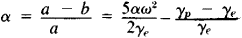# Earth Spheroid

The following article is from The Great Soviet Encyclopedia (1979). It might be outdated or ideologically biased.

## Earth Spheroid

a geometrical figure closely approximating a sphere that is slightly oblate in the direction of the poles and best representing the shape of a geoid, or the shape of the earth as a whole. In the simplest case a spheroid coincides with an ellipsoid of revolution and is the equilibrium shape of a homogeneous fluid mass all of whose particles are mutually attracted according to the law of universal gravitation and which rotates with a constant angular velocity about a fixed axis. Although the earth is not a homogeneous fluid mass, the earth spheroid differs but little from the corresponding ellipsoid of revolution. The deviation of the surface of the earth spheroid from the surface of the earth ellipsoid is greatest—about 3-4 m—at a latitude ot 45°. Consequently, in geodesy the shape of a geoid is usually replaced by an ellipsoid of revolution that has semiaxes of corresponding dimensions and that has a definite position in the body of the earth, and all geodetic problems are solved on the surface of this ellipsoid.

The deviation of a spheroid or ellipsoid from an exact sphere with respect to any planet, including the earth, is characterized by its polar compression α, which is defined by the theory of the French mathematician A. Clairaut (1743) and is equal towhere a and b are the equatorial and polar radii, γe and γp the acceleration of the force of gravity at the equator and the pole, and ω the angular velocity of the planet’s rotation about a fixed axis.

### REFERENCE

Mikhailov, A. A. Kurs gravimetrii i teoriifigury Zemli, 2nd ed. Moscow, 1939.

A. A. IZOTOV

The Great Soviet Encyclopedia, 3rd Edition (1970-1979). © 2010 The Gale Group, Inc. All rights reserved.
Site: Follow: Share:
Open / Close